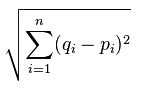## Euclidean Distance theory

Welcome to the 15th part of our Machine Learning with Python tutorial series, where we're currently covering classification with the K Nearest Neighbors algorithm. In the previous tutorial, we covered how to use the K Nearest Neighbors algorithm via Scikit-Learn to achieve 95% accuracy in predicting benign vs malignant tumors based on tumor attributes. Now, we're going to dig into how K Nearest Neighbors works so we have a full understanding of the algorithm itself, to better understand when it will and wont work for us.

We will come back to our breast cancer dataset, using it on our custom-made K Nearest Neighbors algorithm and compare it to Scikit-Learn's, but we're going to start off with some very simple data first. K Nearest Neighbors boils down to proximity, not by group, but by individual points. Thus, all this algorithm is actually doing is computing distance between points, and then picking the most popular class of the top `K` classes of points nearest to it. There are various ways to compute distance on a plane, many of which you can use here, but the most accepted version is Euclidean Distance, named after Euclid, a famous mathematician who is popularly referred to as the father of Geometry, and he definitely wrote the book (The Elements) on it, which is arguably the "bible" for mathematicians. Euclidean distance is:So what's all this business? Basically, it's just the square root of the sum of the distance of the points from eachother, squared. In Python terms, let's say you have something like:

```plot1 = [1,3]
plot2 = [2,5]
euclidean_distance = sqrt( (plot1-plot2)**2 + (plot1-plot2)**2 )```

In this case, the distance is `2.236`.

That's basically the main math behind K Nearest Neighbors right there, now we just need to build a system to handle for the rest of the algorithm, like finding the closest distances, their group, and then voting.

The next tutorial:• Practical Machine Learning Tutorial with Python Introduction

• Regression - Intro and Data

• Regression - Features and Labels

• Regression - Training and Testing

• Regression - Forecasting and Predicting

• Pickling and Scaling

• Regression - Theory and how it works

• Regression - How to program the Best Fit Slope

• Regression - How to program the Best Fit Line

• Regression - R Squared and Coefficient of Determination Theory

• Regression - How to Program R Squared

• Creating Sample Data for Testing

• Classification Intro with K Nearest Neighbors

• Applying K Nearest Neighbors to Data

• Euclidean Distance theory
• Creating a K Nearest Neighbors Classifer from scratch

• Creating a K Nearest Neighbors Classifer from scratch part 2

• Testing our K Nearest Neighbors classifier

• Final thoughts on K Nearest Neighbors

• Support Vector Machine introduction

• Vector Basics

• Support Vector Assertions

• Support Vector Machine Fundamentals

• Constraint Optimization with Support Vector Machine

• Beginning SVM from Scratch in Python

• Support Vector Machine Optimization in Python

• Support Vector Machine Optimization in Python part 2

• Visualization and Predicting with our Custom SVM

• Kernels Introduction

• Why Kernels

• Soft Margin Support Vector Machine

• Kernels, Soft Margin SVM, and Quadratic Programming with Python and CVXOPT

• Support Vector Machine Parameters

• Machine Learning - Clustering Introduction

• Handling Non-Numerical Data for Machine Learning

• K-Means with Titanic Dataset

• K-Means from Scratch in Python

• Finishing K-Means from Scratch in Python

• Hierarchical Clustering with Mean Shift Introduction

• Mean Shift applied to Titanic Dataset

• Mean Shift algorithm from scratch in Python

• Dynamically Weighted Bandwidth for Mean Shift

• Introduction to Neural Networks

• Installing TensorFlow for Deep Learning - OPTIONAL

• Introduction to Deep Learning with TensorFlow

• Deep Learning with TensorFlow - Creating the Neural Network Model

• Deep Learning with TensorFlow - How the Network will run

• Deep Learning with our own Data

• Simple Preprocessing Language Data for Deep Learning

• Training and Testing on our Data for Deep Learning

• 10K samples compared to 1.6 million samples with Deep Learning

• How to use CUDA and the GPU Version of Tensorflow for Deep Learning

• Recurrent Neural Network (RNN) basics and the Long Short Term Memory (LSTM) cell

• RNN w/ LSTM cell example in TensorFlow and Python

• Convolutional Neural Network (CNN) basics

• Convolutional Neural Network CNN with TensorFlow tutorial

• TFLearn - High Level Abstraction Layer for TensorFlow Tutorial

• Using a 3D Convolutional Neural Network on medical imaging data (CT Scans) for Kaggle

• Classifying Cats vs Dogs with a Convolutional Neural Network on Kaggle

• Using a neural network to solve OpenAI's CartPole balancing environment